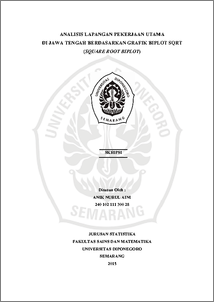# ANALISIS LAPANGAN PEKERJAAN UTAMA DI JAWA TENGAH BERDASARKAN GRAFIK BIPLOT SQRT (SQUARE ROOT BIPLOT)

AINI, ANIK NURUL (2016) ANALISIS LAPANGAN PEKERJAAN UTAMA DI JAWA TENGAH BERDASARKAN GRAFIK BIPLOT SQRT (SQUARE ROOT BIPLOT). Undergraduate thesis, FSM Universitas Diponegoro.Preview
PDF
2861Kb

## Abstract

Biplot analysis is one of the methods of descriptive statistical analysis that can present data of the n objectswhichp variables into a two-dimensional graph. Biplot has several types according to the scale of α used. There are three scales α which is often used in the biplotanalysis, that are α = 0, α = 0,5 and α = 1. Biplot with α = 1 is called the RMP biplot (Row Matric Preserving). Biplot with α = 0 is called CMP biplot (Column Matric Preserving). While biplot with α = 0,5 called SQRT biplot (Square Root Biplot). Biplot with a scale of α = 0,5 is the best biplot to describe a data, because it make a graph between variable and object spread evenly. This study aims to create a SQRT biplot amount of population aged 15 years and over who worked according to district/city and major employment opportunities in Central Java. Biplot chart shows areas that have similar characteristics with the closest Euclidean distance. The diversity of characteristics is indicated by the length of the vector, the longest vector contained in the agricultural sector. Based on the biplot analysis in this study, it was obtained that the goodness size biplot is equal to 64,19958%. Keywords: Biplot, Singular Value Decomposition, Jobs, SQRT, Square Root Biplot

Item Type: Thesis (Undergraduate) H Social Sciences > HA Statistics Faculty of Science and Mathematics > Department of Statistics 49516 Mr Hasbi Yasin 26 Jul 2016 09:01 26 Jul 2016 09:01

Repository Staff Only: item control page